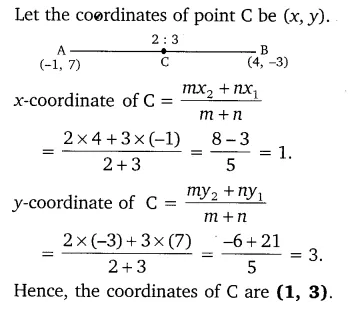# Find the coordinates of the point which divides the join of (-1, 7) and (4, -3)

Find the coordinates of the point which divides the join of (-1, 7) and (4, -3) in the ratio 2 : 3.